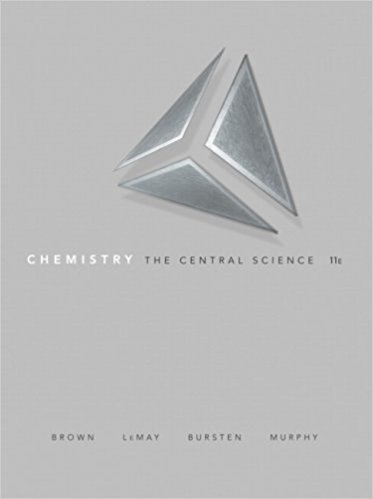×
×

# Solutions for Chapter 3: STOICHIOMETRY: CALCULATIONS WITH CHEMICAL FORMULAS AND EQUATIONS## Full solutions for Chemistry: The Central Science | 11th Edition

ISBN: 9780136006176Solutions for Chapter 3: STOICHIOMETRY: CALCULATIONS WITH CHEMICAL FORMULAS AND EQUATIONS

Solutions for Chapter 3
4 5 0 379 Reviews
13
2
##### ISBN: 9780136006176

Since 98 problems in chapter 3: STOICHIOMETRY: CALCULATIONS WITH CHEMICAL FORMULAS AND EQUATIONS have been answered, more than 21564 students have viewed full step-by-step solutions from this chapter. This expansive textbook survival guide covers the following chapters and their solutions. Chapter 3: STOICHIOMETRY: CALCULATIONS WITH CHEMICAL FORMULAS AND EQUATIONS includes 98 full step-by-step solutions. Chemistry: The Central Science was written by and is associated to the ISBN: 9780136006176. This textbook survival guide was created for the textbook: Chemistry: The Central Science , edition: 11.

Key Chemistry Terms and definitions covered in this textbook
• amplitude.

The vertical distance from the middle of a wave to the peak or trough. (7.1)

• atomic orbital.

The wave function (?) of an electron in an atom. (7.5)

• bar

A unit of pressure equal to 105 Pa. (Section 10.2)

• Basicity

An equilibrium property measured by the position of equilibrium in an acid-base reaction, as, for example, the acid-base reaction between ammonia and water.

• Bonding molecular orbital

A molecular orbital in which electrons have a lower energy than they would in isolated atomic orbitals

• breeder reactor.

A nuclear reactor that produces more fissionable materials than it uses. (19.5)

• crystallization

The process in which molecules, ions, or atoms come together to form a crystalline solid. (Section 13.2)

• Dalton’s law of partial pressures.

The total pressure of a mixture of gases is just the sum of the pressures that each gas would exert if it were present alone. (5.6)

• E

For alkenes, a stereodescriptorthat indicates that the two priority groups are on opposite sides of the p bond.

A form of energy that has wave characteristics and that propagates through a vacuum at the characteristic speed of 3.00 * 108 m >s. (Section 6.1)

• electron configuration

The arrangement of electrons in the orbitals of an atom or molecule (Section 6.8)

• homotopic

Protons that are interchangeable by rotational symmetry.

• nucleic acids

Polymers of high molecular weight that carry genetic information and control protein synthesis. (Section 24.10)

• physical changes

Changes (such as a phase change) that occur with no change in chemical composition. (Section 1.3)

• primary cell

A voltaic cell that cannot be recharged. (Section 20.7)

• quantum mechanics

A mathematical description of an electron that incorporates its wavelike properties.

A compound that prevents a radical chain process from either getting started or continuing.

• substrate

The starting alkyl halide in a substitution or elimination reaction.

• thermodynamic control

A reaction for which the ratio of products is determined solely by the distribution of energy among the products.

• trigonal pyramidal

A geometry adopted by an atom that has one lone pair and a steric number of 4.

×